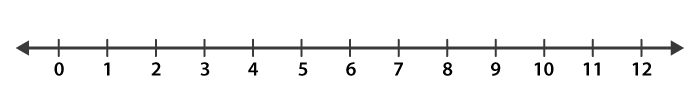# Between which two whole numbers on the number line are the given numbers lie? Which of these whole numbers is nearer the number? (a) 0.8 (b) 5.1 (c) 2.6 (d) 6.4 (e) 9.1 (f) 4.9Solution:

(a) 0.8 lies between the numbers 0 and 1

0.8 is nearer to 1

(b) 5.1 lies between the numbers 5 and 6

5.1 is nearer to 5

(c) 2.6 lies between the numbers 2 and 3

2.6 is nearer to 3

(d) 6.4 lies between the numbers 6 and 7

6.4 is nearer to 6

(e) 9.1 lies between the numbers 9 and 10

9.1 is nearer to 9

(f) 4.9 lies between the numbers 4 and 5

4.9 is nearer to 5(1)(0)